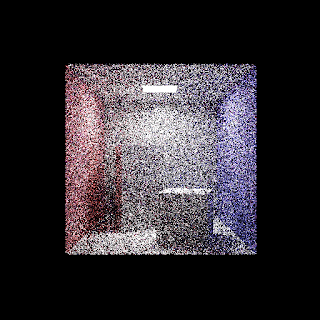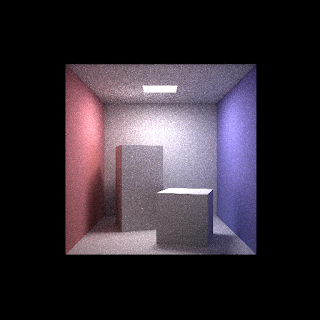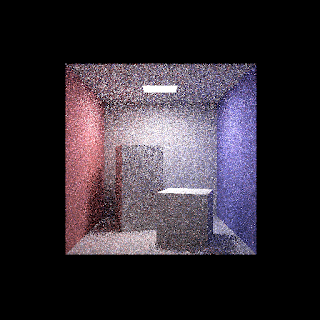### Simple GPU Path Tracer

Introduction
Path tracing is getting more popular in recent years. And because it is easy to get the code run in parallel, so making the path tracer to run on GPU can greatly reduce the rendering time. This post is just my personal notes about learning the basic of Path Tracing and to make me familiar with the D3D12 API. The source code can be downloaded here. And for those who don't want to compile from the source, the executable can be downloaded here.

Rendering Equation
Like other rendering algorithm, path tracing is solving the rendering equation:

To solve this integral, Monte Carlo Integration can be used, so we will shoot many rays within a single pixel from the camera position.

During path tracing, when a ray hits a surface, we can accumulate its light emission as well as the reflected light of that surface, i.e. computing the rendering equation. But we only take one sample in the Monte Carlo Integration so that only 1 random ray is generated according to the surface normal, which simplify the equation to:

Since we shoot many rays within a single pixel, we can still get an un-biased result. To expand the recursive path tracing rendering equation, we can derive the following equation:

GPU random number
To compute the Monte Carlo Integration, we need to generate random number on the GPU. The wang_hash is used due to its simple implementation.
1. uint wang_hash(uint seed)
2. {
3.     seed = (seed ^ 61) ^ (seed >> 16);
4.     seed *= 9;
5.     seed = seed ^ (seed >> 4);
6.     seed *= 0x27d4eb2d;
7.     seed = seed ^ (seed >> 15);
8.     return seed;
9. }
We use the pixel index as the input for the wang_hash function.
seed = px_pos.y * viewportSize.x + px_pos.x
However, there are some visible pattern for the random noise texture using this method (although not affecting the final render result much...):

Luckily, to fix this, we can simply multiple a random number for the pixel index which eliminate the visible pattern in the random texture.
seed = (px_pos.y * viewportSize.x + px_pos.x) * 100

To generate multiple random numbers within the same pixel, we can add the random seed by a constant number after each call to the wang_hash function. Any constant larger than 0, (e.g. 10) will be good enough for this simple path tracer.
1. float rand(inout uint seed)
2. {
3.     float r= wang_hash(seed) * (1.0 / 4294967296.0);
4.     seed+= 10;
5.     return r;
6. }
Scene Storage
To trace ray on the GPU, I upload all the scene data(e.g. triangles, material, light...) into several structure buffers and constant buffer. Due to my laziness and the announcement of DirectX Raytracing, I did not implement any ray tracing acceleration structure like BVH. I just store the triangles in a big buffer.

Tracing Rays
By using the rendering equation derived above, we can start writing code to shoot rays from the camera. During each frame, for each pixel, we trace one ray and reflect it multiple times to compute the rendering equation. And then we can additive blend the path traced result over multiple frames to get a progressive path tracer using the following blend factor:

To generate the random reflected direction of any ray hit surface, we simply uniformly sample a direction on the hemi-sphere around surface normal:

Here is the result of the path tracer when using the uniform random direction and using an emissive light material. The result is quite noisy:Uniform implicit light sampling, 64 sample per pixel

To reduce noise, we can weight the randomly reflected ray with a cosine factor similar to the Lambert diffuse surface:
The result is still a bit noisy. Because in our scene, the light source is not very large, the probability of a randomly reflected ray to hit the light source is quite low. So to improve this, we can explicit sample the light source for every ray that hit a surface.

To sample a rectangular light source, we can randomly choose a point over its surface area, and the corresponding probability will be:
1/area of light
Since our light sampling is over the area domain instead of the direction domain as state in the above equation. The rendering equation need to multiply by the Jacobian that relates solid angle to area. i.e.

With the same number of sample per pixel, the result is much less noisy:Uniform explicit light sampling, 64 sample per pixelCos weighted explicit light sampling, 64 sample per pixel

Simple de-noise

As we have seen above, the result of path tracing is a bit noise even with 64 samples per pixel. The result will be even worse for the first frame:first frame path traced result
There are some very bright dots and looks not good during camera motion. So I added a simple de-noise pass, which is just blurring lots of pixels where they are located on the same surface (which really need a lot of pixel to make the result looks good, which cost some performance...).Blurred first frame path traced result
To identify the pixel correspond to which surface, we store this data in the alpha channel of the path tracing texture with the following formula:
dot(surface_normal, float3(1, 10, 100)) + (mesh_idx + 1) * 1000
This works because we only contains small number of mesh and the mesh normal are the same for each surface in this simple scene.

Random Notes...
During the implementation, I encounter various bugs/artifacts which I think is interesting.

First, is about the simple de-noise pass. It may bleed the light source color to neighbor pixel far away even we have per pixel mesh index data.

This is because we only store a single mesh index per pixel, but we jitter the ray shot from camera within a single pixel per frame, some of the light color will be blend to the light geometry edge. It get very noticeable because the light source have a very high radiance compared to the reflect light of ceiling geometry.

To fix this, I just simply do not jitter the ray for tracing a direct hit of light geometry from camera, so this fix can only apply to explicit light sampling.

The second one is about quantization when using 16bit floating point texture. The path tracing texture sometimes may get quantized result after several hundred frames of additive blend when the single sample per pixel path trace result is very noise.

To work around this, 32bit floating point texture need to be used, but this may have a performance impact (explicitly for my simple de-noise pass...).

The last one is the bright flyflies artifact when using a very large light source (as big as ceiling). This may sound counter intuitive. And the implicit light path traced result(i.e. not sampling the light source directly) does not have those flyflies...

But it turns out this artifact is not related to the size of the light source, but is related to the light too close to the reflected geometry. To visualize it, we may look at how the light get bounced:

The flyflies start to appear in first bound, located at the position near the light source. And then those flyflies get propagated with the reflected light rays. Those large values are generated by explicit light sampling Jacobian transform, the denominator part, which is the distance square between the light and surface.

After a brief search on the internet, to fix this, either need to implement radiance clamping or bi-directional path tracing, or greatly increase the sampling number. Here is the result with over 75000 number of samples per pixel, but it still contains some flyflies...

Conclusion
In this post, we discuss the steps to implement a simple GPU path tracer. The most basic path tracer is simply shooting large number of rays per pixel, and reflect the ray multiple times until it hits a light source. With explicit light sampling, we can greatly reduce noise.

This path tracer is just my personal toy project, which only have Lambert diffuse reflection with a single light. It is my first time to use the D3D12 API, the code is not well optimized, so the source code are for reference only and if you find any bugs, please let me know. Thank you.

Reference
 Physically Based Rendering http://www.pbrt.org/

#### 1 則留言:

1.I did some promo for you

http://mawazo.byethost7.com/viewtopic.php?f=20&t=176&p=181#p181